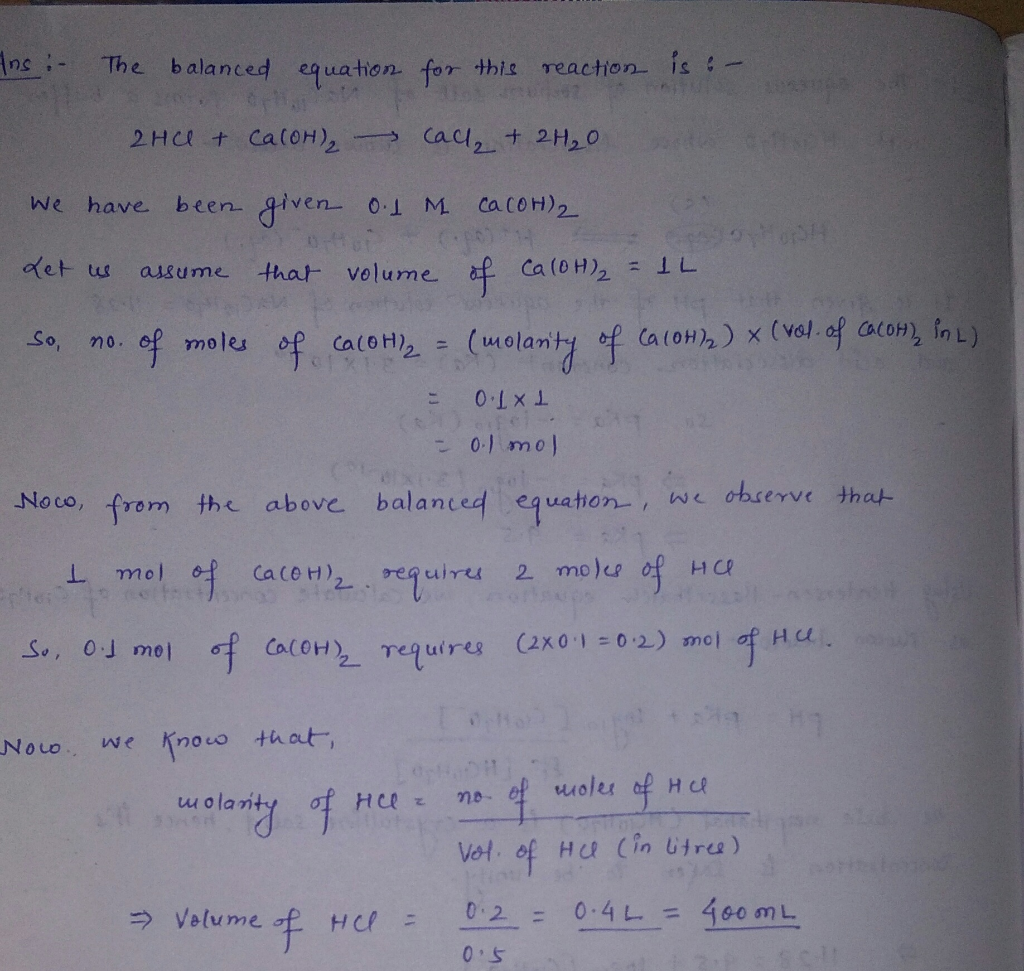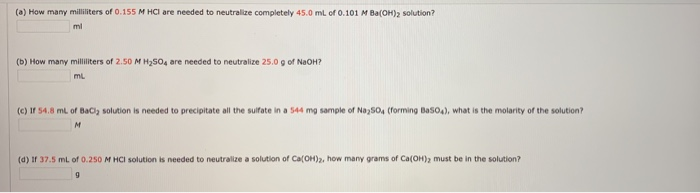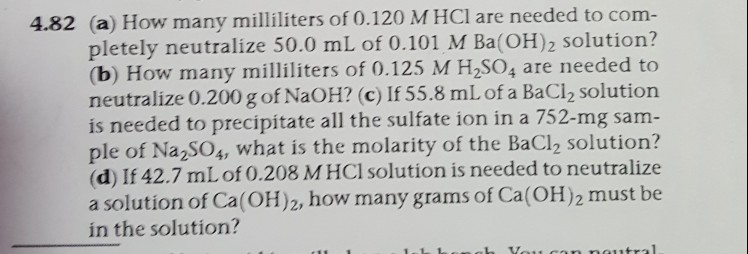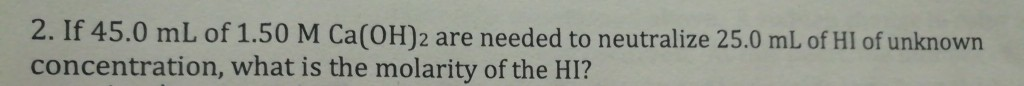Question

What is the volume of 0.5 M HCl needed to neutralize 0.1 M Ca (OH)2?#### Earn Coins

Coins can be redeemed for fabulous gifts.

Similar Homework Help Questions
• ### If 42.4 mL of 0.212 M HCl solution is needed to neutralize a solution of Ca(OH)2, how many grams of Ca(OH)2 must be in t...

If 42.4 mL of 0.212 M HCl solution is needed to neutralize a solution of Ca(OH)2, how many grams of Ca(OH)2 must be in the solution? Express the mass in grams to three significant digits.

• ### What volume of a 0.500 M HCl solution is needed to completely neutralize 10.0 mL of...

What volume of a 0.500 M HCl solution is needed to completely neutralize 10.0 mL of a 0.200 M Ba(OH)2 solution? Ba(OH)2(aq) + 2 HCl(aq) → BaCl2(aq) + 2 H2O(l) a. 10.0 mL b. 4.00 mL c. 2.00 mL d. 8.00 mL

• ### A.How many milliliter of 0.125 M HCl are needed to completely neutralize 58.0 mL of 0.106M...

A.How many milliliter of 0.125 M HCl are needed to completely neutralize 58.0 mL of 0.106M Ba(OH)2 solution? B. How many milliliter of 0.129 M H2SO4 are needed to neutralize 0.150g of NaOH? C. If 56.4mL of Bacl2 solution is needed to precipitate all the sulfate ion in a 748mg sample of na2 so4, what is the molarity of the solution? D. If 43.0ml of 0.208 M HCl solution is needed to neutralize a solution of Ca(OH)2 how many grams...

• ### (0) How many milliers of 0.155 M HCl are needed to neutralize completely 45.0 ml of...(0) How many milliers of 0.155 M HCl are needed to neutralize completely 45.0 ml of 0.101 M Ba(OH)2 solution? ml (b) How many milliliters of 2.50 M H2SO4 are needed to neutralize 25.0 9 of NaOH? (c) If 54.8 ml of BaCl solution is needed to precipitate all the sulfate in a 544 mg sample of Na2SO4 (forming Basod), what is the molarity of the solution? (d) If 37.5 mL of 0.250 M HCl solution is needed to neutralize...

• ### 4.82 (a) How many milliliters of 0.120 M HCI are needed to com- pletely neutralize 50.0 mL of 0.101 M Ba(OH)2 soluti...4.82 (a) How many milliliters of 0.120 M HCI are needed to com- pletely neutralize 50.0 mL of 0.101 M Ba(OH)2 solution? (b) How many milliliters of 0.125 M H2SO4 are needed to neutralize 0.200 g of NaOH? (c) If 55.8 mL of a BaCl2 solution is needed to precipitate all the sulfate ion in a 752-mg sam- ple of Na2SOs, what is the molarity of the BaCl2 solution? (d) If 42.7 mL of 0.208 M HCl solution is needed...

• ### The solubility of slaked lime, Ca(OH)2, in water is 0.185 g/100.0 mL. What volume of 3.85×10-3...

The solubility of slaked lime, Ca(OH)2, in water is 0.185 g/100.0 mL. What volume of 3.85×10-3 M HCl is needed to neutralize 13.5 mL of a saturated Ca(OH)2 solution? The answer is in mL.

• ### What volume of an aqueous 0.25 M solution of HCl is needed to completely neutralize an...

What volume of an aqueous 0.25 M solution of HCl is needed to completely neutralize an aqueous solution of NaOH that has a pH of 12.5 and a volume of 200 mL?

• ### What volume of 0.1292 M Ba(OH)2 would neutralize 50.00 mL of 0.1000 M HCl solution? Ba(OH)2(aq)...

What volume of 0.1292 M Ba(OH)2 would neutralize 50.00 mL of 0.1000 M HCl solution? Ba(OH)2(aq) + 2HCl(aq) ⟶ BaCl2(aq) + 2H2O(l)

• ### How to calculate the volume of 0.025 M Hcl that would completely neutralize 20ml of 0.0125M...

How to calculate the volume of 0.025 M Hcl that would completely neutralize 20ml of 0.0125M Ca(OH)2?

• ### titration: 2. If 45.0 mL of 1.50 M Ca(OH)2 are needed to neutralize 25.0 mL of...titration: 2. If 45.0 mL of 1.50 M Ca(OH)2 are needed to neutralize 25.0 mL of HI of unknown concentration, what is the molarity of the HI? How many mL of 0.100 M NaOH are needed to titrate 20.0 mL of 0.100 M H2SO4?